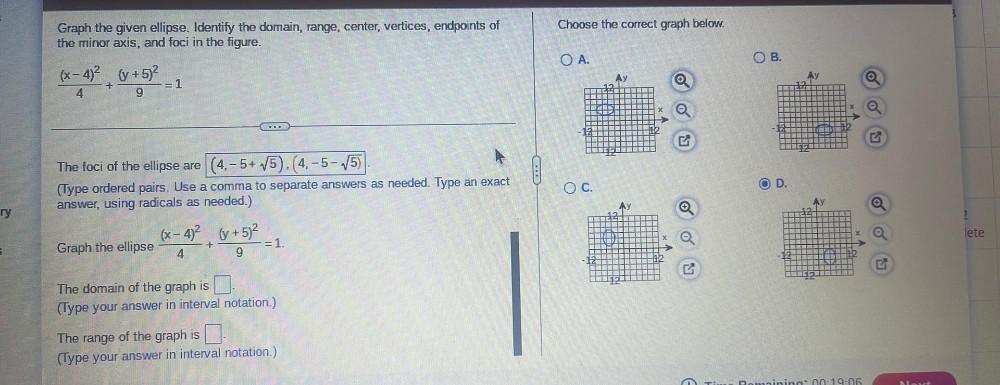Question:

# Choose the correct graph below. Graph the given ellipse. Identify the domain, range, center, vertices, endpoints of the minor axChoose the correct graph below. Graph the given ellipse. Identify the domain, range, center, vertices, endpoints of the minor axis, and foci in the figure. OA. OB. (x-4)2 (+5)2 Ay Ay + =1 4 9 B - ! The foci of the ellipse are (4.-5+ V5).(4.-5-15) (Type ordered pairs. Use a comma to separate answers as needed. Type an exact answer, using radicals as needed.) D. OC. AY ry 2 (x-4)2 (y + 5)2 lete + Graph the ellipse = 1. 4 9 The domain of the graph is (Type your answer in interval notation.) The range of the graph is a (Type your answer in interval notation.)